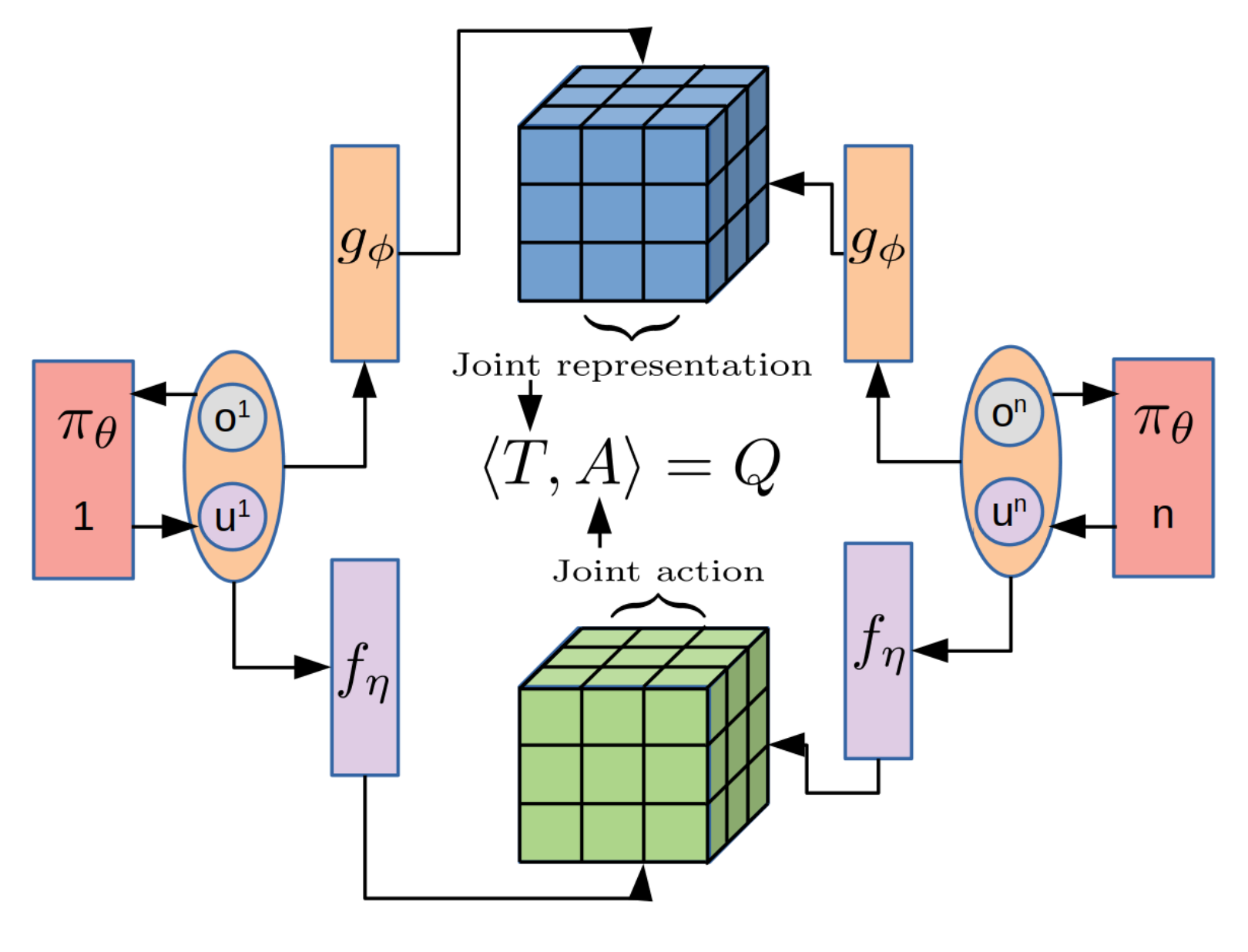Reinforcement Learning in large action spaces is a challenging problem. Cooperative multi-agent reinforcement learning (MARL) exacerbates matters by imposing various constraints on communication and observability. In this work, we consider the fundamental hurdle affecting both value-based and policy-gradient approaches: an exponential blowup of the action space with the number of agents. For value-based methods, it poses challenges in accurately representing the optimal value function. For policy gradient methods, it makes training the critic difficult and exacerbates the problem of the lagging critic. We show that from a learning theory perspective, both problems can be addressed by accurately representing the associated action-value function with a low-complexity hypothesis class. This requires accurately modelling the agent interactions in a sample efficient way. To this end, we propose a novel tensorised formulation of the Bellman equation. This gives rise to our method Tesseract, which views the Q-function as a tensor whose modes correspond to the action spaces of different agents. Algorithms derived from Tesseract decompose the Q-tensor across agents and utilise low-rank tensor approximations to model agent interactions relevant to the task. We provide PAC analysis for Tesseract-based algorithms and highlight their relevance to the class of rich observation MDPs. Empirical results in different domains confirm Tesseract’s gains in sample efficiency predicted by the theory.